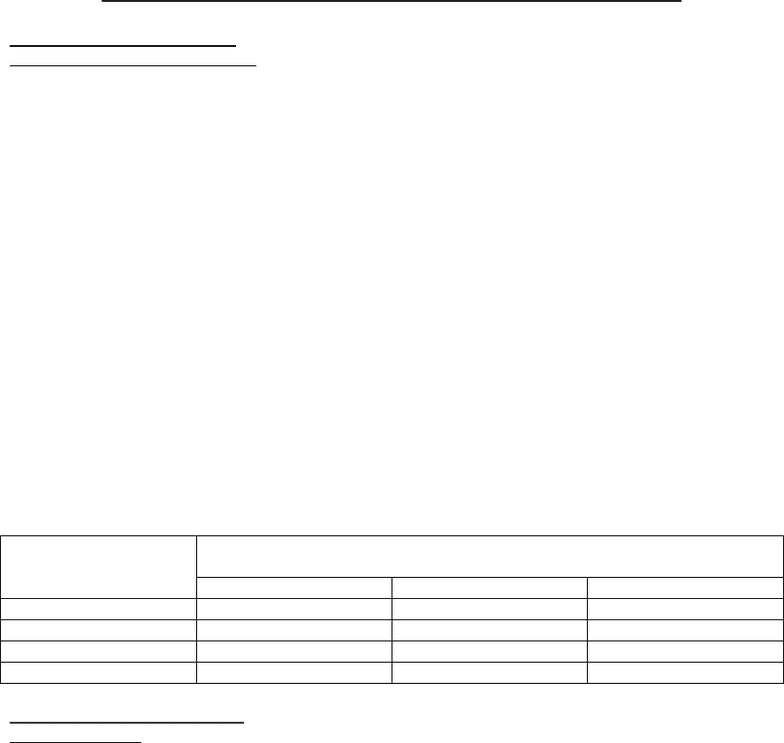# SOAN 2120 Chapter Notes - Chapter 8: Standard Deviation, Descriptive Statistics, Statistical Inference

14 views3 pages
School
Course
ProfessorChapter 8: Analysis of Quantitative Data – page #192-219:
Results With One Variable:
Measures of Central Tendency:
Researchers often want to summarize the information about one variable into a single
number they use three measures of central tendency, or measures of the center of the
frequency distribution: mean, median, and mode, which are often called averages (a less
precise and less clear way of saying the same thing)
The mode is the easiest to use and can be used with nominal, ordinal, interval, or ratio data
it is simply the most common or frequently occurring number
The median is the middle point it is also the 50th percentile, or the point at which half the
cases are above it and half below it; it can be used with ordinal-, interval-, or ratio-level data
(but not nominal level)
o The easiest way is first to organize the scores from highest to lowest, then count to
the middle if there is an odd number of scores, its simple; if there is an even
number of scores the median is somewhere between the two middle numbers
The mean, also called the arithmetic average, is the most widely used measure of central
tendency; it can be used only with interval- or ratio-level data
o Compute the mean by adding up all scores, then divide by the number of scores
If the frequency distributions form a “normal” or bell-shaped curve, the three measures of
central tendency equal each other if the distribution is a skewed distribution, then the three
will not be equal
If most cases have lower scores with a few extreme high scores, the mean will be the
highest, the median in the middle, and the mod the lowest if most cases have higher scores
with a few extreme low scores, the mean will be the lowest, the median in the middle, and the
mode the highest
Level of
Measurement
Measure Of Central
Tendency
Mode
Median
Mean
Nominal
Yes
Ordinal
Yes
Yes
Interval
Yes
Yes
Yes
Ratio
Yes
Yes
Yes
Results with Two Variables:
Bivariate Tables:
What is a Bivariate Table?
The bivariate continency table is widely used it presents the same information as a
scattergram in a more condensed form
The data can be measured at any level of measurement, although interval and ratio data
must be grouped if there are many different values
The table is based on cross-tabulation; that is, the cases are organized in the table on the
basis of two variables at the same time
A contingency table is formed by cross-tabulating two or more variables it is contingent
because the cases in each category of variable get distributed into each category of a second
(or additional) variable
The table distributes cases into the categories of multiple variables at the same time and
shows how the cases, by category of one variable are, “contingent upon” the categories of
other variables
Researchers convert raw count tables into percentaged tables to see bivariate relationships
there are three ways to percentage table: by row, by column, and for the total
1. By row compute the percentage of each cell as a percentage of the row total
2. By column when calculating column percentages, compute the percentage each cell is
the column total
Unlock document

This preview shows page 1 of the document.
Unlock all 3 pages and 3 million more documents.

Already have an account? Log in

# Get access

Grade+
\$10 USD/m
Billed \$120 USD annually
Homework Help
Class Notes
Textbook Notes
40 Verified Answers
Study Guides
1 Booster Class
Class+
\$8 USD/m
Billed \$96 USD annually
Homework Help
Class Notes
Textbook Notes
30 Verified Answers
Study Guides
1 Booster Class